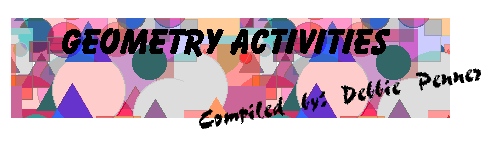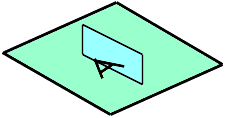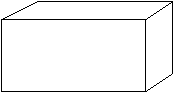## Activity One

1. From a catalogue cut out pictures of different toys.
2. Look carefully at each picture.
3. From construction paper cut out the shape or shapes you see in each toy.
4. Make a chart showing the toys with the shapes beside.
5. What shape do you see the most?

## Activity Two

1. Make a skeleton model of a square using 4 strips of cardboard fastened with paper clips.
2. Holding the bottom rigid, push the top or opposite side sideways
3. What are the characteristics of the new shape?
4. How is this new shape, called a RHOMBUS, diferent from the square?

Solutions:
3. Opposite sides are parallel. Opposite sides are equal.
4. The rhombus does not necessarily have right angles.

## Activity Three

1. Trace a circular region on paper and cut it out.
2. How can you find its center?
3. What other discoveries did you make?

Possible Solutions:
2. Measure across and find midpoint.

## Activity Four

1. In the room find models of each of the following shapes:
• cube
• rectangular prism
• triangular prism
• cylinder
• cone
• sphere

2. Make a chart to show the following information:
• Number of Faces
• Number of Verticals
• Number of Edges
• Number of Curved Surfaces
• Shape of Faces

## Activity Five

1. Colour each of the angles of a triangle a colour.
2. Tear off each angle and fit them together.
3. What discovery did you make?
4. Do the same with several different triangles.
6. What do you notice?

Solutions
3. The angles form a straight line.
4. The same results. The angles of a triangle equal 180 degrees.

## Activity Six

1. Find the sum of all the angles in:
• a triangle
• a pentagon
2. What pattern is established?
3. Can this same pattern be used to calculate the number of degrees in a decagon? or a 16 sided polygon?

Solutions:
1.a) 180 degrees b) 360 degrees c) 540 degrees (three triangles)

2. There are 2 less triangles in the polygons than the number of sides.

3. a)10 - 2=8 trianglesb)8x180=1440 degrees

4. a)16 - 2=14 trianglesb)14x180 degrees=2520 degrees

## Activity Seven

1. Draw 3, 4, 5, 6-sided plane polygons.
2. Draw all diagonals for each shape.
3. Make a chart for each figure to show the following: number of diagonals from each vertex and total no. of diagonals.
4. What is the relationship between the number of sides and the number of diagonals that can be drawn from any vertex of that polygon?
5. How many diagonals can be drawn from any vertex of a 10 sided polygon.

Solutions:PolygonNo. of Diaginalsfrom each VertexTotal No. of DiagonalsTriangle00
Pentagon25
Hexagon39

## Activity Eight

1. Use a square piece of paper.
2. Fold it from one corner to its opposite corner.
3. Cut along this fold. This is called a DIAGONAL.
4. What shape is each part? Are they the same size?
5. Cut along a diagonal of each of the following:
• rectangle
• paralellogram
• rhombus
• regular hexagon
6. Is one part the same shape and size as the other?

Solutions:
4. Each part is a triangle and yes they are the same.
6. Yes, each part is the same.

## Activity Nine

1. Draw the following figures to find the number of intersections that occur when placed on top of each other:
• triangle on triangle
• rectangle on rectangle
• pentagon on pentagon
• hexagon on hexagon
2. What is the maximum number of intersection for each?

Solutions:
1. a) 6 b) 4 c) 10 d) 12
2. Twice the sides

## Activity Ten

1. Use a number of unit squares.
2. Make as many patterns as you can. Remember to use the same number of squares each time.
3. Make a diagram of each pattern on graph paper.
4. Which pattern has the greatest perimeter?
5. Which has the smallest perimeter?

## Activity Eleven

1. Cut irregular shapes from cardboard.
2. Using graph paper, find which shape has the largest area?
3. How did find which has the largest area?

Solutions:
Count the square and half the squares...

## Activity Twelve

1. Make plasticine models of the follwoing solids:
• rectangular prism
• sphere
• cone
• triangluar prism
2. Cut each into two pieces as close the same size as possible.
3. Place one section in front of a mirror.
4. Do you see the complete solid?
5. Cut each solid again, but in a different place.
6. Test with mirror.
7. How many ways can you cut a solid to look complete when put in front of a mirror

Solutions:
4. Yes
7. Once, in half

## Activity Thirteen

1. Obtain printed capital letters of the alphabet.
2. Place a mirror on each letter so that part of the letter and its reflection form the whole letter.For what letters is this possible?
3. For which letters can you place the mirror in more than one position and get this result?

Solutions:
2. A, B, C, D, E, H, I, K M O, T, U, V, W, X, Y
3. H, I, O and X

## Activity Fourteen

1. Select an object shaped like this:2. Find other objects that are the same size and shape as yours.
3. Tell how you checked to find out whether it was the same size and shape.

Hint: Check length, width and angles

## Activity Fifteen

Unscramble some of these geometric words:
• ribsecot
• galen
• reevxt
• gootnac
• niratleg
• cafe
• diusar
• nepal
• rac
• miperteer
• plif
• lehnoydop
• beas
• liequalrate
• noce
Solutions:
bisector
angle
vertex
octagon
triangle
face
pyramid
plane
arc
perimeter
flip
polyhedron
base
equilateral
cone

Go to Math Central